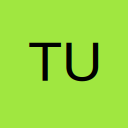# Span overflow text (for a fixed size/font text box) across multiple slides automatically

• Hello - I have a textbox on a slide that I want to have a maximum fixed height (of 6.77 inches...basically to the bottom of the slide). I want the text (regardless of how much text it is) in the box to remain fixed at 44pt. I have a macro which allows the user to replace the text in the box with text from another source (e.g. excel). If the text is relatively short and does not go below the bottom of the slide that's fine and nothing needs to be done. However, if the text is too long (as I want the font size fixed at 44pt) it will go off the visible area of the slide. So I'd like to have a macro which checks if the text has gone off the visible slide (i.e textbox > 6.77 inches), create a new slide (or multiple slides if needed) and 'break up' the text automatically on each slide such that the text box never exceeds the 6.77 inches at 44pt on any given slide and user's text is essentially spanned across slides at a consistent position and size. Does anyone have any suggestions on how to effect this?

My initial thought was to simply check after the text replacement if the text box is greater than 6.77 inches. If so then I'd duplicate the slide (as many times as needed) and delete the relevant portions of text on each of the slides. But in order to delete the text, I'd need to first determine the 'coordinate' where the overflow begins delete all the textafter that point. I'm pretty sure I can work out the mathematical logic to determine the 'coordinate' but I'm just not sure if there is such a property for a vba textbox to have the cursor go to a 'coordinate'. I would assume if there was it would be pretty straightforward at that point to delete all text after that coordinate.

Does anyone have any other thoughts on how to achieve this or may a different approach?